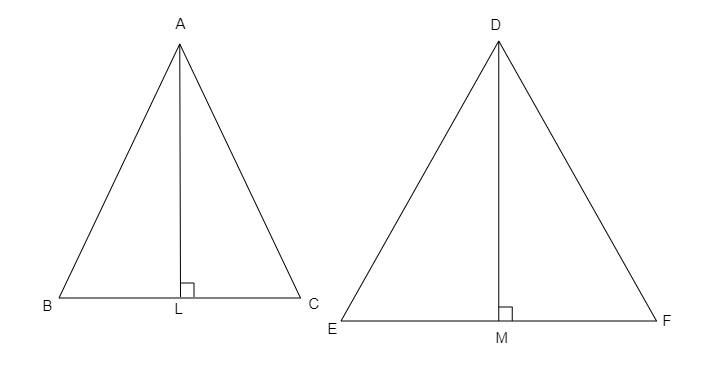# In $\triangle ABC$ and $\triangle DEF$, it is being given that: $AB = 5\ cm, BC = 4\ cm$ and $CA = 4.2\ cm; DE = 10\ cm, EF = 8\ cm$ and $FD = 8.4\ cm$. If $AL \perp BC$ and $DM \perp EF$, find $AL : DM$.

Given:

$AB = 5\ cm, BC = 4\ cm$ and $CA = 4.2\ cm; DE = 10\ cm, EF = 8\ cm$ and $FD = 8.4\ cm$.

$AL \perp BC$ and $DM \perp EF$.
To do:

We have to find $AL : DM$.

Solution:In $\triangle ABC$ and $\triangle DEF$,

$\frac{AB}{DE}=\frac{5}{10}=\frac{1}{2}$

$\frac{AC}{DF}=\frac{4.2}{8.4}=\frac{1}{2}$

$\frac{BC}{EF}=\frac{4}{8}=\frac{1}{2}$

$\frac{AB}{DE}=\frac{AC}{DF}=\frac{BC}{EF}$

Therefore,

By SSS similarity,

$\triangle ABC \sim\ \triangle DEF$

This implies,

$\angle C=\angle F$

In $\triangle ALC$ and $\triangle DMF$,

$\angle ALC=\angle DMF=90^o$

$\angle C=\angle F$

Therefore,

$\triangle ALC \sim\ \triangle DMF$

This implies,

$\frac{AC}{DF}=\frac{AL}{DM}$

$\frac{AL}{DM}=\frac{1}{2}$

$AL:DM=1:2$.

Updated on: 10-Oct-2022

21 Views